# Dilation algorithms – performance of decomposed strels

We looked at the decomposition of different structuring element shapes in my previous post on dilation algorithms. Today let's time some code to how decomposition makes a real difference in performance.

Note that running the code in this post requires that you download my timeit function from the MATLAB Central File Exchange.

For our test image, I'll use a grayscale version of the peppers image that ships with the Image Processing Toolbox.

I = rgb2gray(imread('peppers.png'));
imshow(I)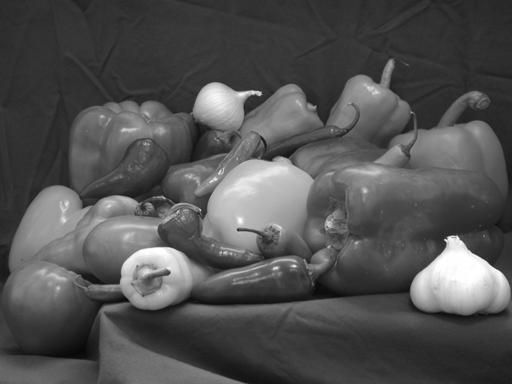Let's time dilations of I with disk structuring elements of varying radii. As I mentioned previously, strel('disk',r) creates a structuring element that is a decomposable approximation to a disk.

% Dilate using radii of 3 through 25.
rr1 = 3:25;

% Initialize the vector that will hold the measured times.
t1 = [];
for r = rr1
se = strel('disk', r);

% Make a function that dilates I by the structuring element se.
f = @() imdilate(I, se);

% Measure how long the dilation takes.
t1(end + 1) = timeit(f);
end

plot(rr1, t1)
grid on
ylabel('Time (seconds)')
% Make sure we show the time scale down to 0.  (Thanks, Chris!)
ylim([0 max(t1)]);
title('Time to compute dilation with decomposable disk')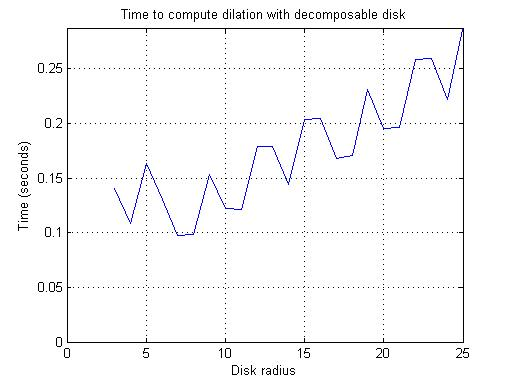Now let's repeat the timing experiment using nondecomposable disks. You make such a structuring element using the syntax strel('disk',r,0).

(In my first draft of this post, I tried to do this step using the same range of radius values as above, 3-25. But I got tired of waiting for it to finish!)

% Dilate using radius values 3-8.
rr2 = 3:8;
t2 = [];
for r = rr2
se = strel('disk', r, 0);
t2(end + 1) = timeit(@() imdilate(I, se));
end

% Plot the results of both experiments together.
plot(rr, t1, rr2, t2)
grid on
ylabel('Time (seconds)')
% Make sure we show the time scale down to 0.  (Thanks, Chris!)
ylim([0 max([t1, t2])]);
legend('Decomposable', 'Nondecomposable');
title('Speed comparison: Decomposable vs. Nondecomposable disks')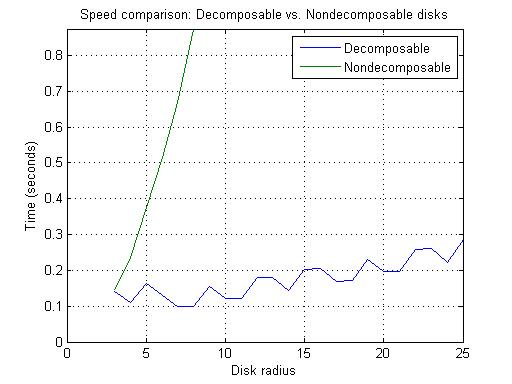I think you can see from the plot above why we chose to use the decomposable disk approximation as the default!

So what does it look like when you dilate gray peppers with a disk?

J = imdilate(I, strel('disk', 25));
imshow(J)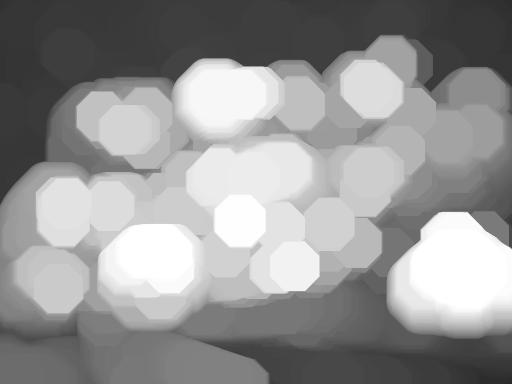The resulting blobs look kind of octagony. That's because of the default decomposition used. You can ask strel to use a closer approximation to a disk. It will take a bit longer to compute, although still not nearly as long as using the nondecomposable disk.

se8 = strel('disk', 25, 8);
J8 = imdilate(I, se8);
imshow(J8)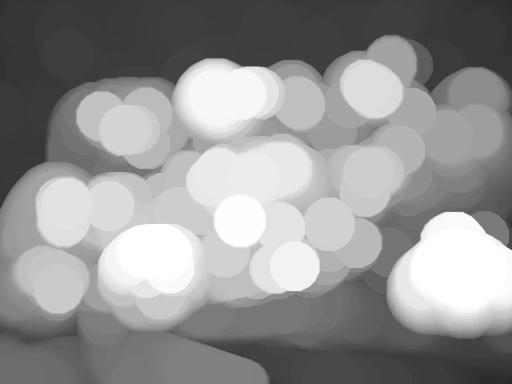Bon appetit!

Published with MATLAB® 7.7

|

### コメント

コメントを残すには、ここ をクリックして MathWorks アカウントにサインインするか新しい MathWorks アカウントを作成します。